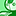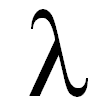# Integration, Extension & APIs

Discussion board where members can learn more about Integration, Extensions and API’s for Qlik Sense.

Announcements
Welcome to Qlik Community! Check out our new navigation! FIND OUT MORE
cancel
Showing results for
Did you mean:Contributor III

## .NET SDK - How can I get a list of fields and measures in the order they appear in the `NxCellRows` object?

Let's say I add a couple of Dimensions and Measures to my HyperCube.  I'm using AddInlineDimension and AddInlineMeasure from here: https://github.com/kolsrud/qlik-dot-net-sdk-hypercube-usage/blob/master/HypercubeUsage/Program.cs

var hyperCube = new HyperCubeDef() { AlwaysFullyExpanded = true };

AddInlineMeasure(hyperCube, "Only([Gross Principal Balance])");

AddInlineDimension(hyperCube, "Status"); // dimension added after measure

In memory, I have a List<T> keeping track of the measures and dimensions I added.  So when I iterate over the pages and rows, I expect the order that I added them would be the same in each `NxCellRows` inside a `NxDataPage` matrix.  I'm finding out this isn't the case...  Dimensions are moved to the front; measures are moved towards the end.

var firstPage = new[] { new NxPage() { Top = 0, Left = 0, Width = 4, Height = 10 } };

foreach(var page in pager.IteratePages(firstPage, Pager.Next)) {

foreach(var row in page.Single().Matrix) {

for(var i = 0; i < columns.Count; ++i) {

// List<T> containing a list of dims/measures added

var cell = row // <-- cell does not match column index ???

}

}

}

1 Solution

Accepted SolutionsEmployee

Actually... I see that the column order property is actually there, but not part of the SDK documentation. You could write like this:

```hypercube.ColumnOrder = new[] { 0, 1, 3, 2 };
```
4 RepliesMaster

Hi,

Qlik keeps two arrays, one with dimensions and one with measures, and normally dimensions comes first in the data rows, and measures ater. You can use the qColumnOrder property in the HyperCube to change this, but I don't know how ggood support for that is in the .Net SDK.

Erik Wetterberg

https://extendingqlik.upper88.com/Contributor III
Author

Hmm. Based on your answer, this is what I ended up doing.  Wondering if there is a better way?

// Fill in dimensions first

var dimensions = hyperCube_.Dimensions.ToList();

for(var i = 0; i < dimensions.Count; ++i)

{

var dimension = dimensions;

var cell = row;

}

// Then fill in the measures

var measures = hyperCube_.Measures.ToList();

for(var j = dimensions.Count; j < (dimensions.Count + measures.Count); ++j)

{

var measure = measures;

var cell = row;

}Employee

If you set the following property of your hypercube, then the data should be returned in the order you expect:

```hypercube.Set("qColumnOrder", new[] { 0, 1, 3, 2 });

```

0, 1 and 2 will refer to the three dimensions (they have indexes starting from 0), and 3 will refer to your measure (measures have indexes starting from number of dimensions.Employee

Actually... I see that the column order property is actually there, but not part of the SDK documentation. You could write like this:

```hypercube.ColumnOrder = new[] { 0, 1, 3, 2 };
```